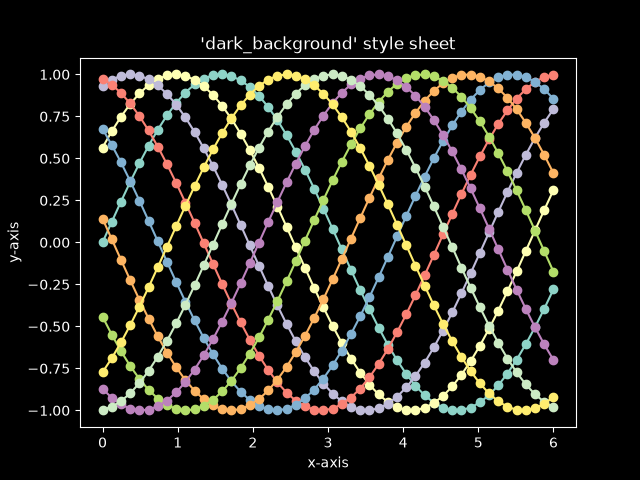# Dark background style sheet#

This example demonstrates the "dark_background" style, which uses white for elements that are typically black (text, borders, etc). Note that not all plot elements default to colors defined by an rc parameter.import matplotlib.pyplot as plt
import numpy as np

plt.style.use('dark_background')

fig, ax = plt.subplots()

L = 6
x = np.linspace(0, L)
ncolors = len(plt.rcParams['axes.prop_cycle'])
shift = np.linspace(0, L, ncolors, endpoint=False)
for s in shift:
ax.plot(x, np.sin(x + s), 'o-')
ax.set_xlabel('x-axis')
ax.set_ylabel('y-axis')
ax.set_title("'dark_background' style sheet")

plt.show()


Gallery generated by Sphinx-Gallery